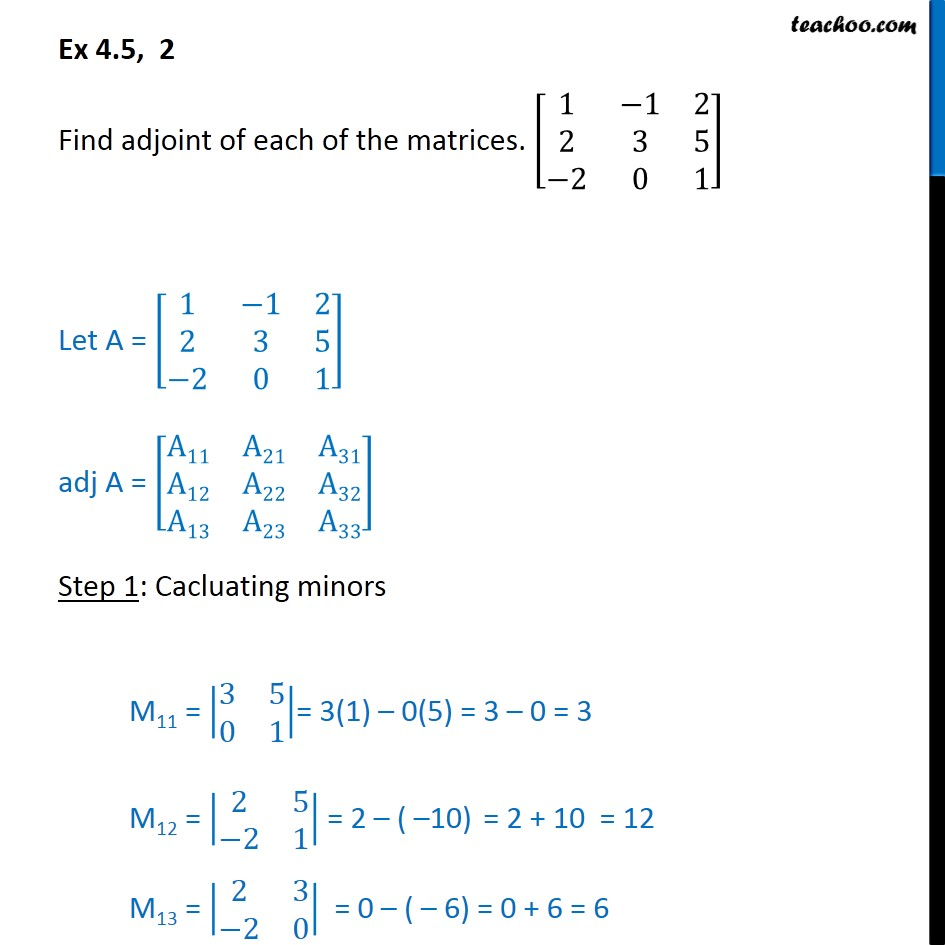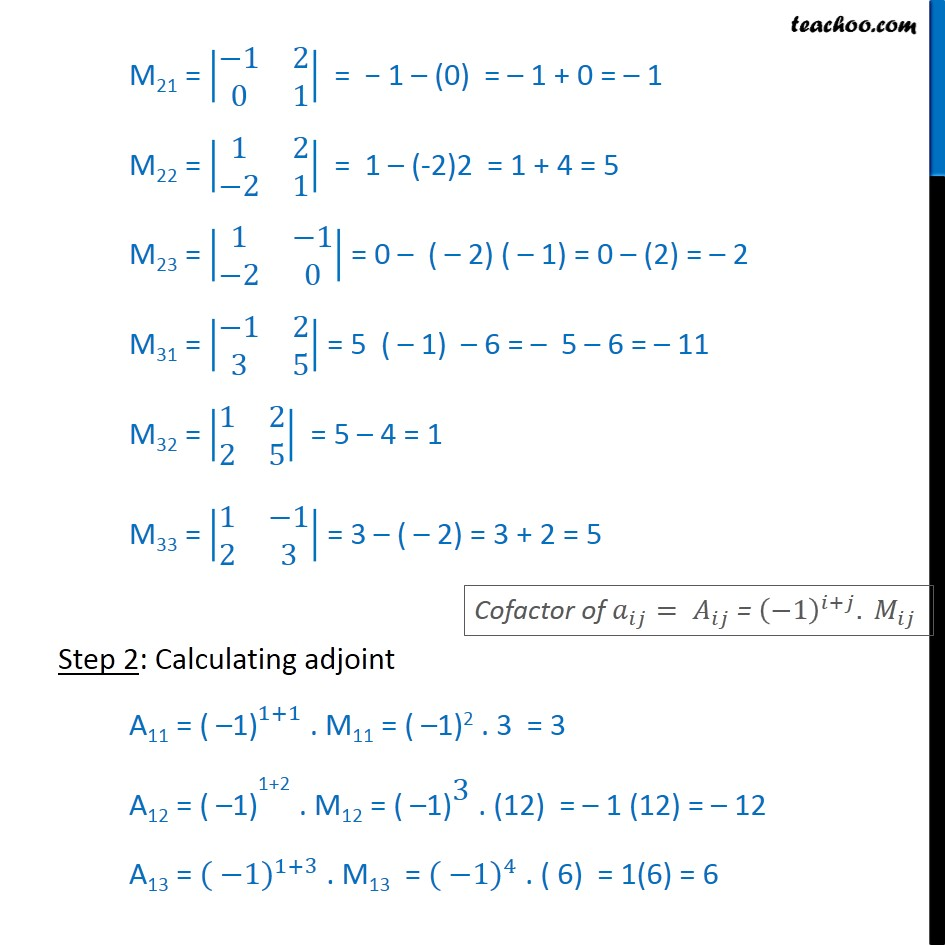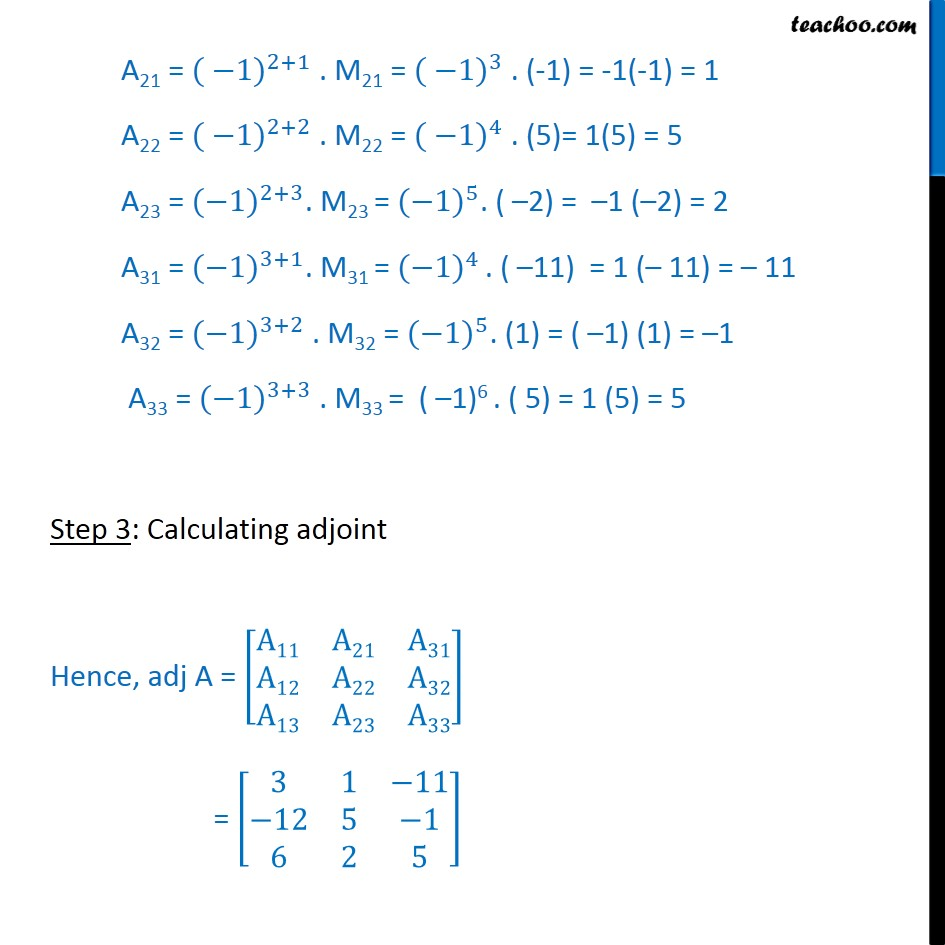Chapter 4 Class 12 Determinants
Concept wiseIntroducing your new favourite teacher - Teachoo Black, at only ₹83 per month

### Transcript

Ex 4.5, 2 Find adjoint of each of the matrices. 1 1 2 2 3 5 2 0 1 Let A = 1 1 2 2 3 5 2 0 1 adj A = A11 A21 A31 A12 A22 A32 A13 A23 A33 Step 1: Cacluating minors M11 = 3 5 0 1 = 3(1) 0(5) = 3 0 = 3 M12 = 2 5 2 1 = 2 ( 10) = 2 + 10 = 12 M13 = 2 3 2 0 = 0 ( 6) = 0 + 6 = 6 M21 = 1 2 0 1 = 1 (0) = 1 + 0 = 1 M22 = 1 2 2 1 = 1 (-2)2 = 1 + 4 = 5 M23 = 1 1 2 0 = 0 ( 2) ( 1) = 0 (2) = 2 M31 = 1 2 3 5 = 5 ( 1) 6 = 5 6 = 11 M32 = 1 2 2 5 = 5 4 = 1 M33 = 1 1 2 3 = 3 ( 2) = 3 + 2 = 5 Step 2: Calculating adjoint A11 = ( 1) 1+1 . M11 = ( 1)2 . 3 = 3 A12 = ( 1) 1+2 . M12 = ( 1) 3 . (12) = 1 (12) = 12 A13 = ( 1) 1+3 . M13 = ( 1) 4 . ( 6) = 1(6) = 6 A21 = ( 1) 2+1 . M21 = ( 1) 3 . (-1) = -1(-1) = 1 A22 = ( 1) 2+2 . M22 = ( 1) 4 . (5)= 1(5) = 5 A23 = ( 1) 2+3 . M23 = ( 1) 5 . ( 2) = 1 ( 2) = 2 A31 = ( 1) 3+1 . M31 = ( 1) 4 . ( 11) = 1 ( 11) = 11 A32 = ( 1) 3+2 . M32 = ( 1) 5 . (1) = ( 1) (1) = 1 A33 = ( 1) 3+3 . M33 = ( 1)6 . ( 5) = 1 (5) = 5 Step 3: Calculating adjoint Hence, adj A = A11 A21 A31 A12 A22 A32 A13 A23 A33 = 3 1 11 12 5 1 6 2 5